Courses

# Partnership - Quantitative Aptitude Quant Notes | EduRev

## Quant : Partnership - Quantitative Aptitude Quant Notes | EduRev

The document Partnership - Quantitative Aptitude Quant Notes | EduRev is a part of the Quant Course Quantitative Aptitude for Competitive Examinations.
All you need of Quant at this link: Quant

PARTNERSHIP

When two or more than two persons run a business jointly, they are called partners and the deal is known as a partnership.

CONCEPT OF PARTNERSHIP

1. When investments of all the partners are for the same time, the gain or loss is distributed among the partners in the ratio of their investments.
2.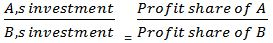Type Of questions Asked For :

1. Share in total profit
2. Profit ratio
3. Time
4. Capital

Ex: In a business A, B and C are invested Rs. 380, Rs. 400, and 420 respectively. Divide a net profit of Rs. 180 among the partners.

Sol: A’s profit: B’s profit: C’s profit = A : B : C
= 380 : 400 : 420
= 19 : 20 : 21

Profit share of A =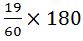= 57 Rs.

Profit share of B =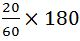= 60 Rs.

Profit share of C =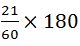= 63 Rs.

Ex: A, B and C started a business by investing Rs. 120000 Rs. 135000 and Rs. 150000 respectively. If the difference between the profit of A & B is Rs 2100. Find the total profit.

Sol: Ratio of shares of A, B & C = Ratio of their investment
A : B : C = 120000 : 135000 : 150000
= 8 : 9 : 10
Difference b/w (A & B) = 9 - 8 = 1
1 = 2100
Total profit (8+9+10) = 27
= 27 x 2100
= Rs 56700

Ex: P, Q, and R started a business with investing their money in the ratio of 1 : 2 : 4, after 6 months P invested the half amount more as before and Q invested twice the amount as before while R withdrew 1/4th of their investments. Find the ratio of their profits at the end of the year?
Sol:
Ratio = P : Q : R

= (1 x 6 +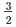x 6) : (2 x 6 + 4 x 6) : (4 x 6 + 3 x 6)
= 5 : 12 : 14

Ex: Two companies A and B entered into a partnership just 5 months ago. The ratio of profit claimed by A and B is 6 : 17. If B had just started his business 12 months ago with Rs.1275, what is the amount contributed by A?

Sol: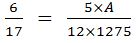A =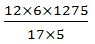= Rs.1080

Ex: A, B and C start a business each investing Rs. 20,000. After 5 months A withdraws Rs. 5000, B withdraws Rs. 4000 and C invest Rs. 6000 more. At the end of the year, the total profit of Rs. 69,900. Find the share of each.

Sol: Ratio of the capitals of A, B and C.

= (20,000 × 5 + 15000 × 7) : (20000 × 5 + 16000 × 7) : (20000 × 5 + 26000×7)
= 20,5000 : 212000 : 282000
= 205 : 212 : 282
A’s share = 69900 ×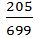= 20500 Rs
B’s share = 69900 ×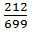= 21200 Rs
C’s share = 69900 ×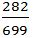= 28200 Rs

Ex: A invested Rs. 76000 in a business. After few months, B joined him with Rs. 57000. At the end of the year, the total profit was divided between them in the ratio 2 : 1. After how many months did B join ?

Sol: Let B joined after x months.

Then B’s money was invested for (12 – x) months
A B
76000 x 12 : 57000 x (12 – x)
76 x 12 : 57 (12 – x )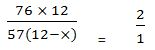912 = 114 (12 - x)
8 = 12 - x
x = 4

Hence, B joined after 4 months.

Ex: A and B are partners in a business. They invest in the ratio of 5 : 6, at the end of 8 months A withdraws. If they receive profits in the ratio of 5 : 9, find how long B's investment was used?

Sol:
ratio = A : B
= 5 x 8 : 6 x t
according to the question,
5 x 8 : 6 x t = 5 : 9
t = 12 months

Ex: In a partnership, A invests of 1/6 the capital for 1/6 of the time, B invests 1/3 of the capital for 1/3 of the time and C, the rest of the capital for whole time. Find A’s share out of the total profit of Rs. 2300.

Sol: Capital of C = 1 -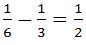Let the total time be 1 year.

A’s profit : B’s profit : C’s profit = A : B : C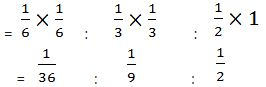= 1 : 4 : 18

Share of A =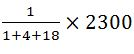= Rs. 100

Ex.: A is working and B is a sleeping partner in a business. A puts in Rs.5,000 and B puts in Rs.6,000. A receives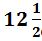% of the profit for managing the business and the rest is divided in proportion of their capitals. What does each get out of a profit of Rs.880?

Sol: The amount which A receives for managing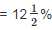of Rs.880 =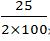×880 = Rs.110
The amount left = 880 – 110 = Rs.770
The amount left is to be divided in the ratio = 5,000 : 6,000 = 5:6
Out of the amount left, A’s share =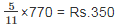Out of the amount left, B’s share =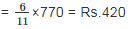Total share receive by A = 110 + 350 = Rs.460
Share received by B = Rs.420

Ex.: A, B and C invested capitals in the ratio of 2:3:5. At the end of the business terms, they received the profit in the ratio of 5:3:12. Find the ratio of time for which they contributed their capitals ?

Sol: Here, P1: P2: P3 = 5 : 3 : 12

and x1: x2: x3= 2 : 3 : 5

The required ratio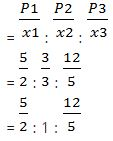=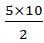: 1×10: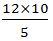= 25 : 10 : 24

Ex.: A starts a business with Rs.4000 and B joins the business 4 months later with an investment of Rs.5000. After a year, they earn a profit of Rs.22000. Find the shares of A and B.

Sol: A’s share : B’s Share
= 4000 × 12 : 5000 × (12 - 4)
= 4 × 12 : 5 × 8 = 6 : 5
Now, let the share of A = 6x
and the share of B = 5x
According to the question,
6x + 5x = 22000
11x = 22000
x = Rs. 2000
Share of A = 6x = 6 × 2000 = Rs. 12000
and share of B = 5x = 5 × 2000 = Rs. 10000

Ex.: In a business partnership among A,B,C and D, the profit is shared as follows.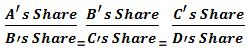= 1/3. If the total profit is Rs.400000, the share of C is-

Sol: Given,
A : B = 1 : 3
B : C = 1 : 3
C : D = 1 : 3
A : B : C : D = 1 × 1 × 1 : 3 × 1 × 1 : 3 × 3 × 1 : 3 × 3 × 3 = 1 : 3 : 9 : 27
Let the profits of A,B,C and D are x, 3x, 9x and 27x respectively.
Partnership of C =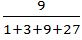× 400000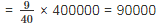Ex.: Alok, Bhism and Chandra hired a meadow jointly for the whole year. Alok put in 80 buffaloes for 10 months, Bhism 100 buffaloes for 5 months and Chandra 150 buffaloes for 4 months. If Alok paid Rs.320 as rent, what is the total rent for the meadow?

Sol: Number of buffaloes put by Alok for 1 month
= 10 × 80 = 800
Number of buffaloes put by Bhisma for 1 month
= 5 × 100 = 500
and number of buffaloes put by Chandra for 1 month
= 4 × 150 = 600
Ratio = 800 : 500 : 600
= 8 : 5 : 6
Sum of ratios = 8 + 5 + 6 = 19
Total rent for the meadow =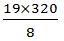= Rs. 760

Ex.: A, B and C started a business by investing Rs.40500, Rs.45,000 and Rs.60,000, respectively. After 6 months C withdrew Rs.15,000 while A invested Rs.4500 more. In annual profit of Rs.56,100 the share of C will exceed that of A by?
Sol: Ratio of equivalent capitals of A, B and C for 1 month
(40500 × 6 + 45000 × 6) : (45000 × 12)
: (60000 × 6 + 45000 × 6)
(405 + 450) : (450 × 2) : (600 + 450)
= 855 : 900 : 1050
= 171 : 180 : 210
= 57 : 60 : 70
Sum of ratios = 57 + 60 + 70 = 187
Required difference =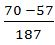× 56100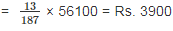Offer running on EduRev: Apply code STAYHOME200 to get INR 200 off on our premium plan EduRev Infinity!

## Quantitative Aptitude for Competitive Examinations

34 videos|34 docs|143 tests

,

,

,

,

,

,

,

,

,

,

,

,

,

,

,

,

,

,

,

,

,

;